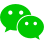• NumPy 基本操作

共1个章节

### 实验任务

• 了解 NumPy 数值类型与数据结构
• 使用 NumPy 对数组进行运算
• 使用 NumPy 对数组进行切片
• 使用 NumPy 对数组形状进行操作
• 使用 NumPy 对数组进行统计

• 本课程难度为简单。

### 面向用户

• 本课程主要讲解 NumPy 的基本操作；该课程适合于对数据分析感兴趣，或者想要使用 Python 进行数据分析的用户。加入社群加入编程交流社群